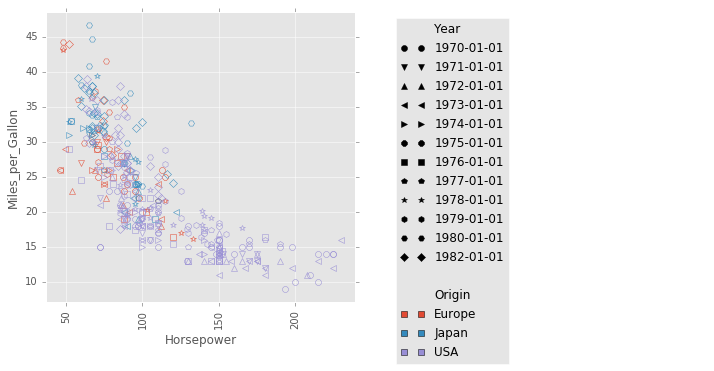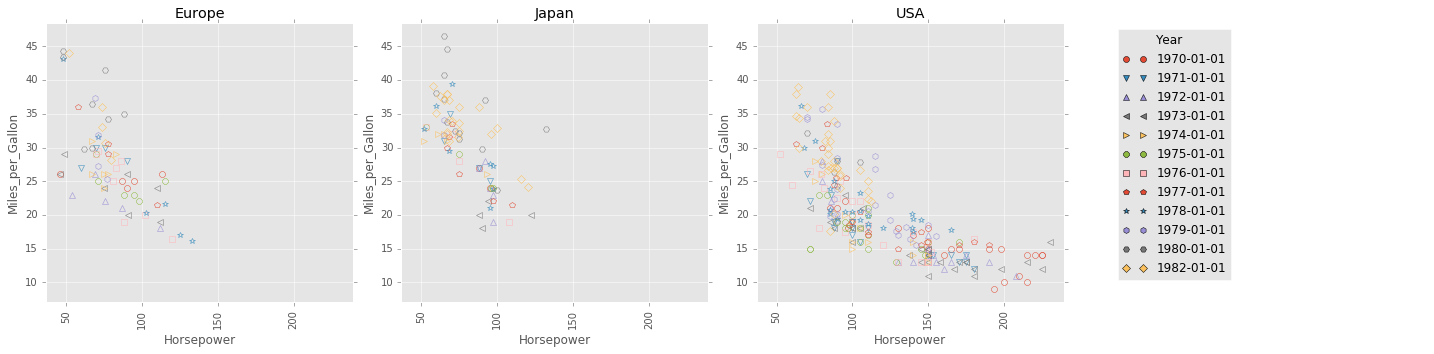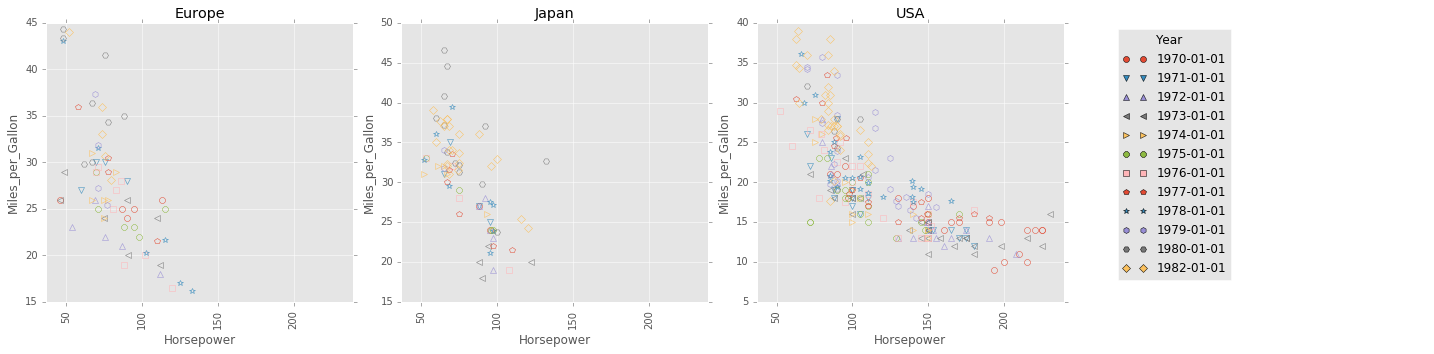# Welcome to matplotlib-helpers’s documentation!¶

## Grid plot¶

The matplotlib_helpers.chart.encode() function is inspired by the altair project.

With matplotlib_helpers.chart.encode() (quoted from the altair documentation):

• The data source is a DataFrame that consists of columns of different data types (quantitative, ordinal, nominal and date/time).
• The DataFrame is in a tidy format where the rows correspond to samples and the columns correspond the observed variables.
• The data is mapped to the visual properties (position, color, size, shape, faceting, etc.) using the group-by operation of Pandas.

## Usage¶

The examples below plot vehicle fuel economy (in miles per gallon) versus horsepower for a dataset from the altair project.

Set marker color by the Year column and set the shape of the each marker according to the Origin column:

from altair import load_dataset
import matplotlib as mpl
import matplotlib.style
import matplotlib_helpers as mplh
import matplotlib_helpers.chart

# load data as a pandas DataFrame

with mpl.style.context(['ggplot']):
mplh.chart.encode(cars,
x='Horsepower',
y='Miles_per_Gallon',
shape='Year',
color='Origin',
cell_size=5, fill=False)Split plot into multiple subplots, with the subplot in each column corresponding to a distinct value in the Origin column.

The same type of handling can be applied using the row keyword.

with mpl.style.context(['ggplot']):
mplh.chart.encode(cars,
x='Horsepower',
y='Miles_per_Gallon',
color='Year',
shape='Year',
column='Origin',
cell_size=5, fill=False)By default, all plots share the same x axis scale and y axis scale. This behaviour can be changed by setting the sharexscale keyword argument or the shareyscale keyword argument.

For example, note that the subplots below all have different x axis and y axis scales.

with mpl.style.context(['ggplot']):
mplh.chart.encode(cars,
x='Horsepower',
y='Miles_per_Gallon',
color='Year',
shape='Year',
column='Origin',
sharexscale=False,
shareyscale=False,
cell_size=5, fill=False)See the matplotlib_helpers.chart.encode() documentation for more details.

Contents: